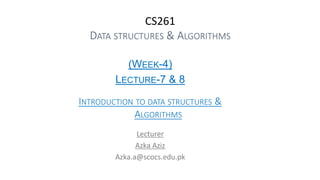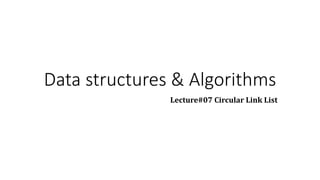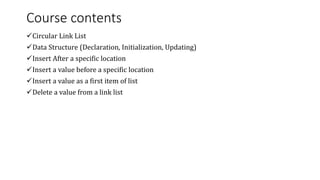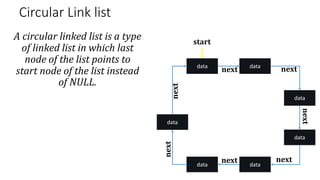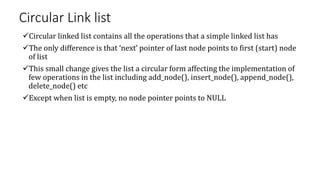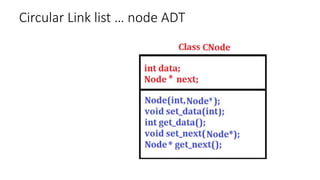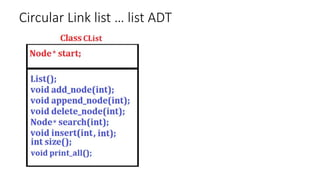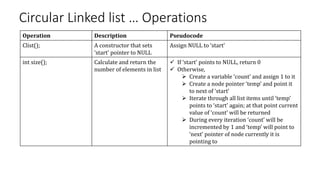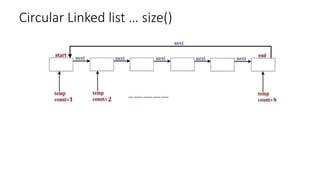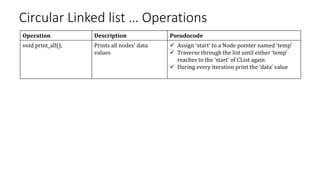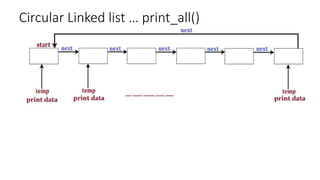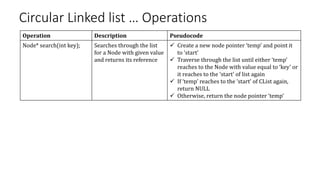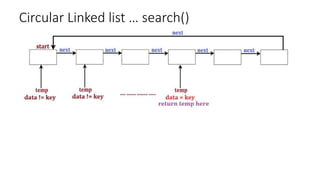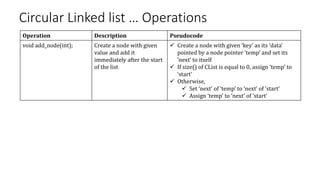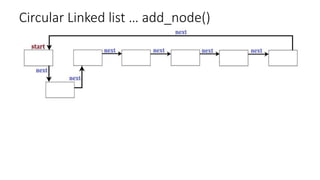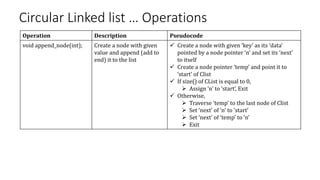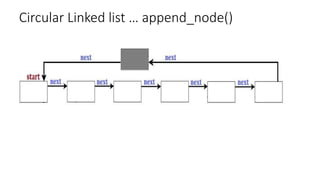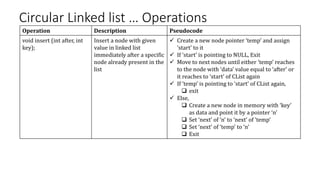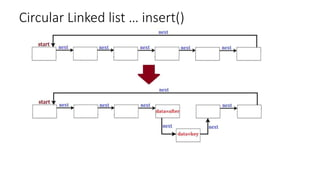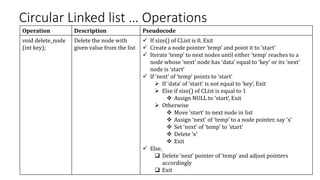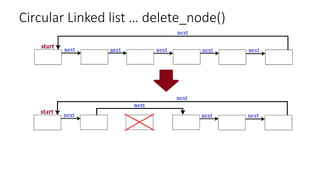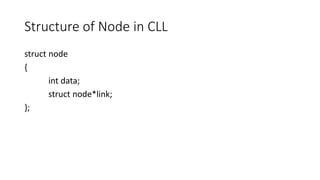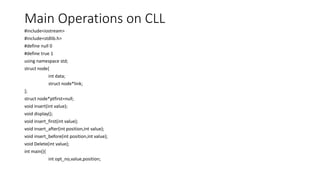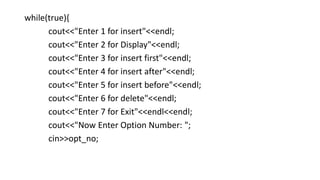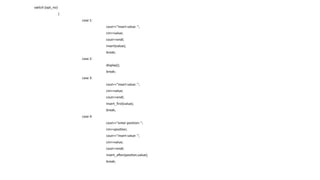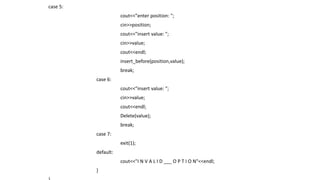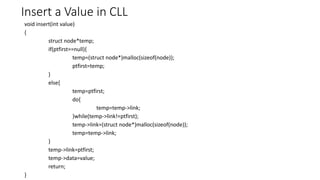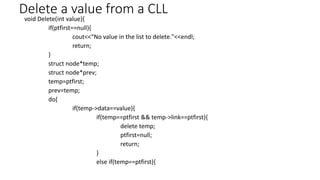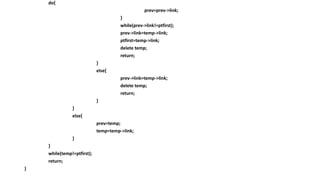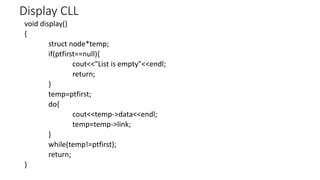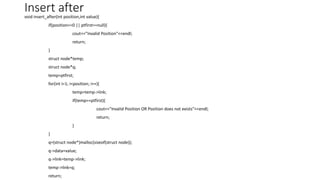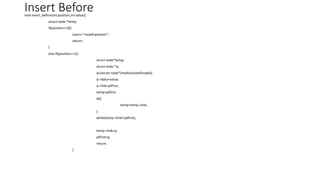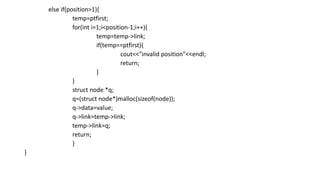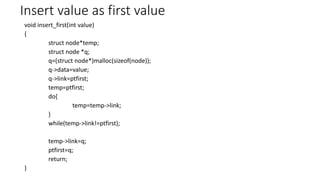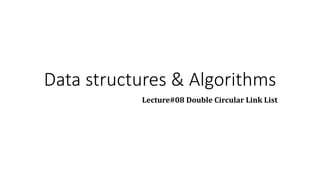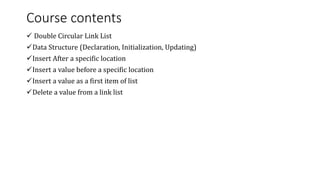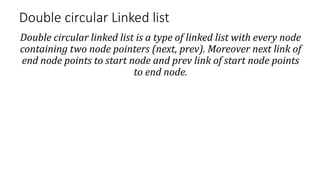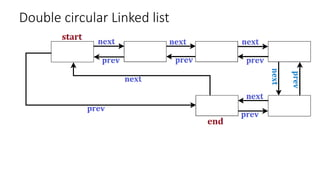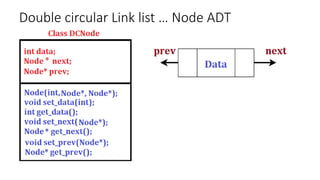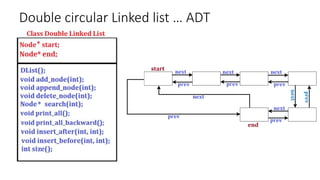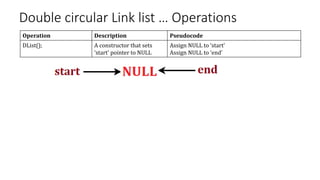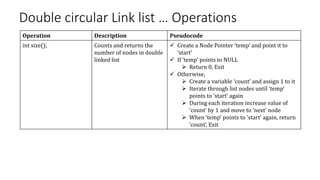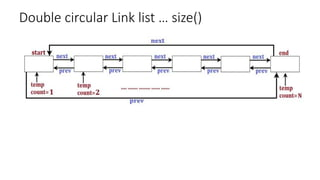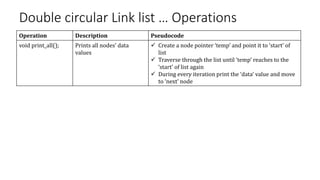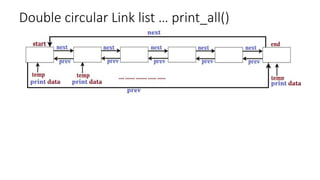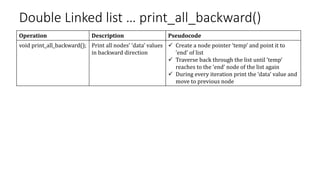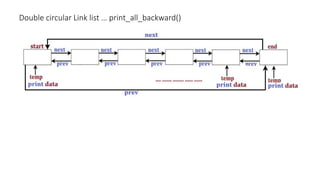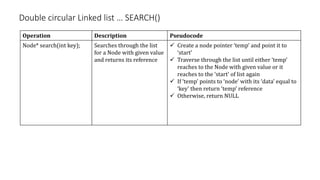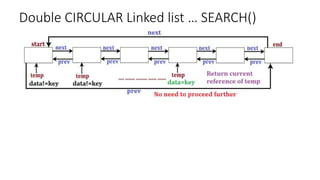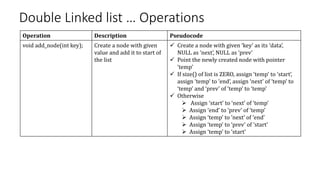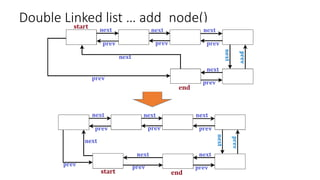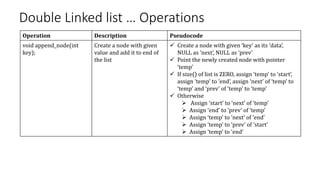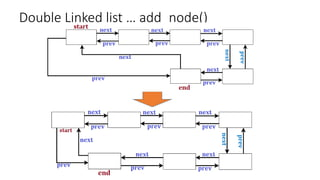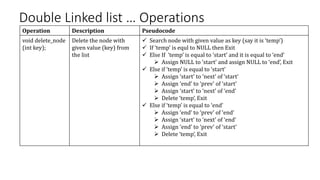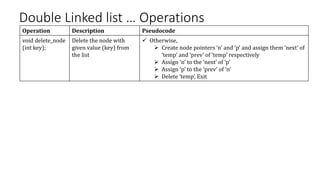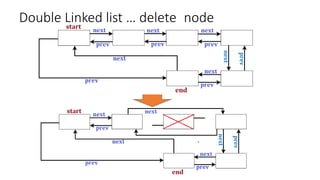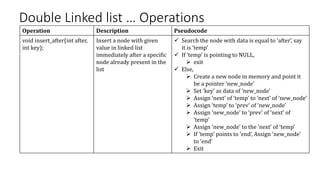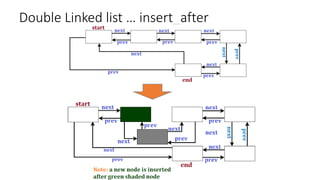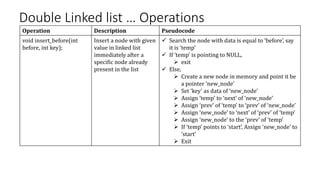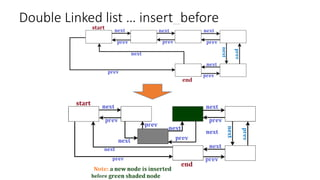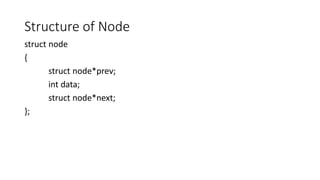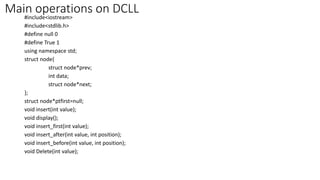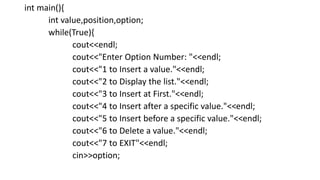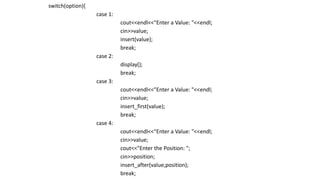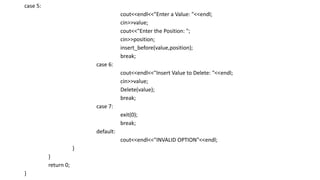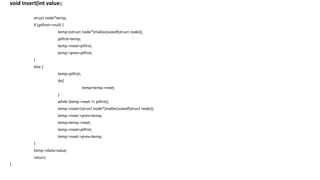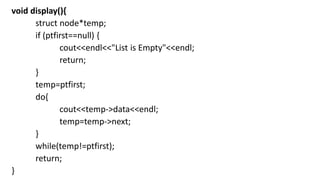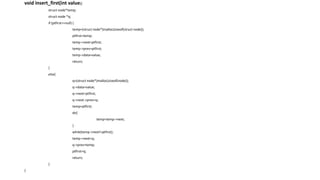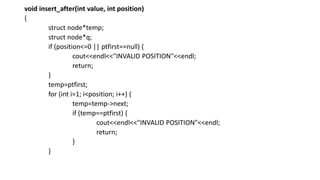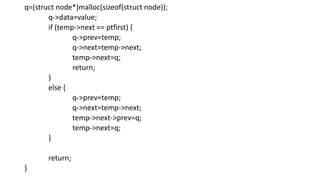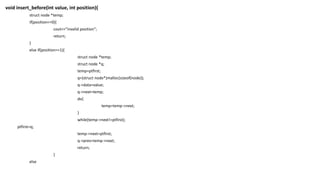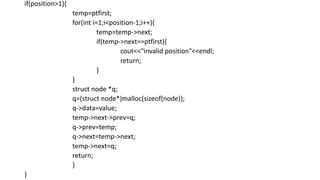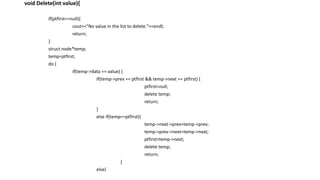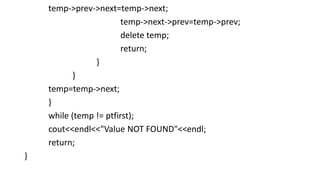1 of 74

### Data structure.pptx

1. CS261 DATA STRUCTURES & ALGORITHMS (WEEK-4) LECTURE-7 & 8 INTRODUCTION TO DATA STRUCTURES & ALGORITHMS Lecturer Azka Aziz Azka.a@scocs.edu.pk
2. Data structures & Algorithms Lecture#07 Circular Link List
3. Course contents Circular Link List Data Structure (Declaration, Initialization, Updating) Insert After a specific location Insert a value before a specific location Insert a value as a first item of list Delete a value from a link list
4. Circular Link list A circular linked list is a type of linked list in which last node of the list points to start node of the list instead of NULL. data data data data data data data start next next next next next next next
5. Circular Link list Circular linked list contains all the operations that a simple linked list has The only difference is that ‘next’ pointer of last node points to first (start) node of list This small change gives the list a circular form affecting the implementation of few operations in the list including add_node(), insert_node(), append_node(), delete_node() etc Except when list is empty, no node pointer points to NULL
8. Circular Linked list … Operations Operation Description Pseudocode Clist(); A constructor that sets ‘start’ pointer to NULL Assign NULL to ‘start’ int size(); Calculate and return the number of elements in list  If ‘start’ points to NULL, return 0  Otherwise,  Create a variable ‘count’ and assign 1 to it  Create a node pointer ‘temp’ and point it to next of ‘start’  Iterate through all list items until ‘temp’ points to ‘start’ again; at that point current value of ‘count’ will be returned  During every iteration ‘count’ will be incremented by 1 and ‘temp’ will point to ‘next’ pointer of node currently it is pointing to
9. Circular Linked list … size()
10. Circular Linked list … Operations Operation Description Pseudocode void print_all(); Prints all nodes’ data values  Assign ‘start’ to a Node pointer named ‘temp’  Traverse through the list until either ‘temp’ reaches to the ‘start’ of CList again  During every iteration print the ‘data’ value
11. Circular Linked list … print_all()
12. Circular Linked list … Operations Operation Description Pseudocode Node* search(int key); Searches through the list for a Node with given value and returns its reference  Create a new node pointer ‘temp’ and point it to ‘start’  Traverse through the list until either ‘temp’ reaches to the Node with value equal to ‘key’ or it reaches to the ‘start’ of list again  If ‘temp’ reaches to the ‘start’ of CList again, return NULL  Otherwise, return the node pointer ‘temp’
13. Circular Linked list … search()
14. Circular Linked list … Operations Operation Description Pseudocode void add_node(int); Create a node with given value and add it immediately after the start of the list  Create a node with given ‘key’ as its ‘data’ pointed by a node pointer ‘temp’ and set its ‘next’ to itself  If size() of CList is equal to 0, assign ‘temp’ to ‘start’  Otherwise,  Set ‘next’ of ‘temp’ to ‘next’ of ‘start’  Assign ‘temp’ to ‘next’ of ‘start’
16. Circular Linked list … Operations Operation Description Pseudocode void append_node(int); Create a node with given value and append (add to end) it to the list  Create a node with given ‘key’ as its ‘data’ pointed by a node pointer ‘n’ and set its ‘next’ to itself  Create a node pointer ‘temp’ and point it to ‘start’ of Clist  If size() of CList is equal to 0,  Assign ‘n’ to ‘start’, Exit  Otherwise,  Traverse ‘temp’ to the last node of Clist  Set ‘next’ of ‘n’ to ‘start’  Set ‘next’ of ‘temp’ to ‘n’  Exit
17. Circular Linked list … append_node()
18. Circular Linked list … Operations Operation Description Pseudocode void insert (int after, int key); Insert a node with given value in linked list immediately after a specific node already present in the list  Create a new node pointer ‘temp’ and assign ‘start’ to it  If ‘start’ is pointing to NULL, Exit  Move to next nodes until either ‘temp’ reaches to the node with ‘data’ value equal to ‘after’ or it reaches to ‘start’ of CList again  If ‘temp’ is pointing to ‘start’ of CList again,  exit  Else,  Create a new node in memory with ‘key’ as data and point it by a pointer ‘n’  Set ‘next’ of ‘n’ to ‘next’ of ‘temp’  Set ‘next’ of ‘temp’ to ‘n’  Exit
19. Circular Linked list … insert()
20. Circular Linked list … Operations Operation Description Pseudocode void delete_node (int key); Delete the node with given value from the list  If size() of CList is 0, Exit  Create a node pointer ‘temp’ and point it to ‘start’  Iterate ‘temp’ to next nodes until either ‘temp’ reaches to a node whose ‘next’ node has ‘data’ equal to ‘key’ or its ‘next’ node is ‘start’  If ‘next’ of ‘temp’ points to ‘start’  If ‘data’ of ‘start’ is not equal to ‘key’, Exit  Else if size() of CList is equal to 1  Assign NULL to ‘start’, Exit  Otherwise  Move ‘start’ to next node in list  Assign ‘next’ of ‘temp’ to a node pointer, say ‘x’  Set ‘next’ of ‘temp’ to ‘start’  Delete ‘x’  Exit  Else,  Delete ‘next’ pointer of ‘temp’ and adjust pointers accordingly  Exit
21. Circular Linked list … delete_node()
22. Structure of Node in CLL struct node { int data; struct node*link; };
23. Main Operations on CLL #include<iostream> #include<stdlib.h> #define null 0 #define true 1 using namespace std; struct node{ int data; struct node*link; }; struct node*ptfirst=null; void insert(int value); void display(); void insert_first(int value); void insert_after(int position,int value); void insert_before(int position,int value); void Delete(int value); int main(){ int opt_no,value,position;
24. while(true){ cout<<"Enter 1 for insert"<<endl; cout<<"Enter 2 for Display"<<endl; cout<<"Enter 3 for insert first"<<endl; cout<<"Enter 4 for insert after"<<endl; cout<<"Enter 5 for insert before"<<endl; cout<<"Enter 6 for delete"<<endl; cout<<"Enter 7 for Exit"<<endl<<endl; cout<<"Now Enter Option Number: "; cin>>opt_no;
25. switch (opt_no) { case 1: cout<<"insert value: "; cin>>value; cout<<endl; insert(value); break; case 2: display(); break; case 3: cout<<"insert value: "; cin>>value; cout<<endl; insert_first(value); break; case 4: cout<<"enter position: "; cin>>position; cout<<"insert value: "; cin>>value; cout<<endl; insert_after(position,value); break;
26. case 5: cout<<"enter position: "; cin>>position; cout<<"insert value: "; cin>>value; cout<<endl; insert_before(position,value); break; case 6: cout<<"insert value: "; cin>>value; cout<<endl; Delete(value); break; case 7: exit(1); default: cout<<"I N V A L I D ___ O P T I O N"<<endl; }
27. Insert a Value in CLL void insert(int value) { struct node*temp; if(ptfirst==null){ temp=(struct node*)malloc(sizeof(node)); ptfirst=temp; } else{ temp=ptfirst; do{ temp=temp->link; }while(temp->link!=ptfirst); temp->link=(struct node*)malloc(sizeof(node)); temp=temp->link; } temp->link=ptfirst; temp->data=value; return; }
28. Delete a value from a CLL void Delete(int value){ if(ptfirst==null){ cout<<"No value in the list to delete."<<endl; return; } struct node*temp; struct node*prev; temp=ptfirst; prev=temp; do{ if(temp->data==value){ if(temp==ptfirst && temp->link==ptfirst){ delete temp; ptfirst=null; return; } else if(temp==ptfirst){
30. Display CLL void display() { struct node*temp; if(ptfirst==null){ cout<<"List is empty"<<endl; return; } temp=ptfirst; do{ cout<<temp->data<<endl; temp=temp->link; } while(temp!=ptfirst); return; }
31. Insert after void insert_after(int position,int value){ if(position<=0 || ptfirst==null){ cout<<"Invalid Position"<<endl; return; } struct node*temp; struct node*q; temp=ptfirst; for(int i=1; i<position; i++){ temp=temp->link; if(temp==ptfirst){ cout<<"Invalid Position OR Position does not exists"<<endl; return; } } q=(struct node*)malloc(sizeof(struct node)); q->data=value; q->link=temp->link; temp->link=q; return;
32. Insert Before void insert_before(int position,int value){ struct node *temp; if(position<=0){ cout<<"invalid position"; return; } else if(position==1){ struct node*temp; struct node *q; q=(struct node*)malloc(sizeof(node)); q->data=value; q->link=ptfirst; temp=ptfirst; do{ temp=temp->link; } while(temp->link!=ptfirst); temp->link=q; ptfirst=q; return; }
33. else if(position>1){ temp=ptfirst; for(int i=1;i<position-1;i++){ temp=temp->link; if(temp==ptfirst){ cout<<"invalid position"<<endl; return; } } struct node *q; q=(struct node*)malloc(sizeof(node)); q->data=value; q->link=temp->link; temp->link=q; return; } }
34. Insert value as first value void insert_first(int value) { struct node*temp; struct node *q; q=(struct node*)malloc(sizeof(node)); q->data=value; q->link=ptfirst; temp=ptfirst; do{ temp=temp->link; } while(temp->link!=ptfirst); temp->link=q; ptfirst=q; return; }
35. Data structures & Algorithms Lecture#08 Double Circular Link List
36. Course contents  Double Circular Link List Data Structure (Declaration, Initialization, Updating) Insert After a specific location Insert a value before a specific location Insert a value as a first item of list Delete a value from a link list
37. Double circular Linked list Double circular linked list is a type of linked list with every node containing two node pointers (next, prev). Moreover next link of end node points to start node and prev link of start node points to end node.
41. Double circular Link list … Operations Operation Description Pseudocode DList(); A constructor that sets ‘start’ pointer to NULL Assign NULL to ‘start’ Assign NULL to ‘end’
42. Double circular Link list … Operations Operation Description Pseudocode int size(); Counts and returns the number of nodes in double linked list  Create a Node Pointer ‘temp’ and point it to ‘start’  If ‘temp’ points to NULL  Return 0, Exit  Otherwise,  Create a variable ‘count’ and assign 1 to it  Iterate through list nodes until ‘temp’ points to ‘start’ again  During each iteration increase value of ‘count’ by 1 and move to ‘next’ node  When ‘temp’ points to ‘start’ again, return ‘count’, Exit
43. Double circular Link list … size()
44. Double circular Link list … Operations Operation Description Pseudocode void print_all(); Prints all nodes’ data values  Create a node pointer ‘temp’ and point it to ‘start’ of list  Traverse through the list until ‘temp’ reaches to the ‘start’ of list again  During every iteration print the ‘data’ value and move to ‘next’ node
45. Double circular Link list … print_all()
46. Double Linked list … print_all_backward() Operation Description Pseudocode void print_all_backward(); Print all nodes’ ‘data’ values in backward direction  Create a node pointer ‘temp’ and point it to ‘end’ of list  Traverse back through the list until ‘temp’ reaches to the ‘end’ node of the list again  During every iteration print the ‘data’ value and move to previous node
47. Double circular Link list … print_all_backward()
48. Double circular Linked list … SEARCH() Operation Description Pseudocode Node* search(int key); Searches through the list for a Node with given value and returns its reference  Create a node pointer ‘temp’ and point it to ‘start’  Traverse through the list until either ‘temp’ reaches to the Node with given value or it reaches to the ‘start’ of list again  If ‘temp’ points to ‘node’ with its ‘data’ equal to ‘key’ then return ‘temp’ reference  Otherwise, return NULL
49. Double CIRCULAR Linked list … SEARCH()
50. Double Linked list … Operations Operation Description Pseudocode void add_node(int key); Create a node with given value and add it to start of the list  Create a node with given ‘key’ as its ‘data’, NULL as ‘next’, NULL as ‘prev’  Point the newly created node with pointer ‘temp’  If size() of list is ZERO, assign ‘temp’ to ‘start’, assign ‘temp’ to ‘end’, assign ‘next’ of ‘temp’ to ‘temp’ and ‘prev’ of ‘temp’ to ‘temp’  Otherwise  Assign ‘start’ to ‘next’ of ‘temp’  Assign ‘end’ to ‘prev’ of ‘temp’  Assign ‘temp’ to ‘next’ of ‘end’  Assign ‘temp’ to ‘prev’ of ‘start’  Assign ‘temp’ to ‘start’
52. Double Linked list … Operations Operation Description Pseudocode void append_node(int key); Create a node with given value and add it to end of the list  Create a node with given ‘key’ as its ‘data’, NULL as ‘next’, NULL as ‘prev’  Point the newly created node with pointer ‘temp’  If size() of list is ZERO, assign ‘temp’ to ‘start’, assign ‘temp’ to ‘end’, assign ‘next’ of ‘temp’ to ‘temp’ and ‘prev’ of ‘temp’ to ‘temp’  Otherwise  Assign ‘start’ to ‘next’ of ‘temp’  Assign ‘end’ to ‘prev’ of ‘temp’  Assign ‘temp’ to ‘next’ of ‘end’  Assign ‘temp’ to ‘prev’ of ‘start’  Assign ‘temp’ to ‘end’
54. Double Linked list … Operations Operation Description Pseudocode void delete_node (int key); Delete the node with given value (key) from the list  Search node with given value as key (say it is ‘temp’)  If ‘temp’ is equl to NULL then Exit  Else If ‘temp’ is equal to ‘start’ and it is equal to ‘end’  Assign NULL to ‘start’ and assign NULL to ‘end’, Exit  Else if ‘temp’ is equal to ‘start’  Assign ‘start’ to ‘next’ of ‘start’  Assign ‘end’ to ‘prev’ of ‘start’  Assign ‘start’ to ‘next’ of ‘end’  Delete ‘temp’, Exit  Else if ‘temp’ is equal to ‘end’  Assign ‘end’ to ‘prev’ of ‘end’  Assign ‘start’ to ‘next’ of ‘end’  Assign ‘end’ to ‘prev’ of ‘start’  Delete ‘temp’, Exit
55. Double Linked list … Operations Operation Description Pseudocode void delete_node (int key); Delete the node with given value (key) from the list  Otherwise,  Create node pointers ‘n’ and ‘p’ and assign them ‘next’ of ‘temp’ and ‘prev’ of ‘temp’ respectively  Assign ‘n’ to the ‘next’ of ‘p’  Assign ‘p’ to the ‘prev’ of ‘n’  Delete ‘temp’, Exit
56. Double Linked list … delete_node
57. Double Linked list … Operations Operation Description Pseudocode void insert_after(int after, int key); Insert a node with given value in linked list immediately after a specific node already present in the list  Search the node with data is equal to ‘after’, say it is ‘temp’  If ‘temp’ is pointing to NULL,  exit  Else,  Create a new node in memory and point it be a pointer ‘new_node’  Set ‘key’ as data of ‘new_node’  Assign ‘next’ of ‘temp’ to ‘next’ of ‘new_node’  Assign ‘temp’ to ‘prev’ of ‘new_node’  Assign ‘new_node’ to ‘prev’ of ‘next’ of ‘temp’  Assign ‘new_node’ to the ‘next’ of ‘temp’  If ‘temp’ points to ‘end’, Assign ‘new_node’ to ‘end’  Exit
58. Double Linked list … insert_after
59. Double Linked list … Operations Operation Description Pseudocode void insert_before(int before, int key); Insert a node with given value in linked list immediately after a specific node already present in the list  Search the node with data is equal to ‘before’, say it is ‘temp’  If ‘temp’ is pointing to NULL,  exit  Else,  Create a new node in memory and point it be a pointer ‘new_node’  Set ‘key’ as data of ‘new_node’  Assign ‘temp’ to ‘next’ of ‘new_node’  Assign ‘prev’ of ‘temp’ to ‘prev’ of ‘new_node’  Assign ‘new_node’ to ‘next’ of ‘prev’ of ‘temp’  Assign ‘new_node’ to the ‘prev’ of ‘temp’  If ‘temp’ points to ‘start’, Assign ‘new_node’ to ‘start’  Exit
60. Double Linked list … insert_before
61. Structure of Node struct node { struct node*prev; int data; struct node*next; };
62. Main operations on DCLL #include<iostream> #include<stdlib.h> #define null 0 #define True 1 using namespace std; struct node{ struct node*prev; int data; struct node*next; }; struct node*ptfirst=null; void insert(int value); void display(); void insert_first(int value); void insert_after(int value, int position); void insert_before(int value, int position); void Delete(int value);
63. int main(){ int value,position,option; while(True){ cout<<endl; cout<<"Enter Option Number: "<<endl; cout<<"1 to Insert a value."<<endl; cout<<"2 to Display the list."<<endl; cout<<"3 to Insert at First."<<endl; cout<<"4 to Insert after a specific value."<<endl; cout<<"5 to Insert before a specific value."<<endl; cout<<"6 to Delete a value."<<endl; cout<<"7 to EXIT"<<endl; cin>>option;
64. switch(option){ case 1: cout<<endl<<"Enter a Value: "<<endl; cin>>value; insert(value); break; case 2: display(); break; case 3: cout<<endl<<"Enter a Value: "<<endl; cin>>value; insert_first(value); break; case 4: cout<<endl<<"Enter a Value: "<<endl; cin>>value; cout<<"Enter the Position: "; cin>>position; insert_after(value,position); break;
65. case 5: cout<<endl<<"Enter a Value: "<<endl; cin>>value; cout<<"Enter the Position: "; cin>>position; insert_before(value,position); break; case 6: cout<<endl<<"Insert Value to Delete: "<<endl; cin>>value; Delete(value); break; case 7: exit(0); break; default: cout<<endl<<"INVALID OPTION"<<endl; } } return 0; }
66. void insert(int value){ struct node*temp; if (ptfirst==null) { temp=(struct node*)malloc(sizeof(struct node)); ptfirst=temp; temp->next=ptfirst; temp->prev=ptfirst; } else { temp=ptfirst; do{ temp=temp->next; } while (temp->next != ptfirst); temp->next=(struct node*)malloc(sizeof(struct node)); temp->next->prev=temp; temp=temp->next; temp->next=ptfirst; temp->next->prev=temp; } temp->data=value; return; }
67. void display(){ struct node*temp; if (ptfirst==null) { cout<<endl<<"List is Empty"<<endl; return; } temp=ptfirst; do{ cout<<temp->data<<endl; temp=temp->next; } while(temp!=ptfirst); return; }
68. void insert_first(int value){ struct node*temp; struct node *q; if (ptfirst==null) { temp=(struct node*)malloc(sizeof(struct node)); ptfirst=temp; temp->next=ptfirst; temp->prev=ptfirst; temp->data=value; return; } else{ q=(struct node*)malloc(sizeof(node)); q->data=value; q->next=ptfirst; q->next->prev=q; temp=ptfirst; do{ temp=temp->next; } while(temp->next!=ptfirst); temp->next=q; q->prev=temp; ptfirst=q; return; } }
69. void insert_after(int value, int position) { struct node*temp; struct node*q; if (position<=0 || ptfirst==null) { cout<<endl<<"INVALID POSITION"<<endl; return; } temp=ptfirst; for (int i=1; i<position; i++) { temp=temp->next; if (temp==ptfirst) { cout<<endl<<"INVALID POSITION"<<endl; return; } }
70. q=(struct node*)malloc(sizeof(struct node)); q->data=value; if (temp->next == ptfirst) { q->prev=temp; q->next=temp->next; temp->next=q; return; } else { q->prev=temp; q->next=temp->next; temp->next->prev=q; temp->next=q; } return; }
71. void insert_before(int value, int position){ struct node *temp; if(position<=0){ cout<<"invalid position"; return; } else if(position==1){ struct node *temp; struct node *q; temp=ptfirst; q=(struct node*)malloc(sizeof(node)); q->data=value; q->next=temp; do{ temp=temp->next; } while(temp->next!=ptfirst); ptfirst=q; temp->next=ptfirst; q->prev=temp->next; return; } else
72. if(position>1){ temp=ptfirst; for(int i=1;i<position-1;i++){ temp=temp->next; if(temp->next==ptfirst){ cout<<"invalid position"<<endl; return; } } struct node *q; q=(struct node*)malloc(sizeof(node)); q->data=value; temp->next->prev=q; q->prev=temp; q->next=temp->next; temp->next=q; return; } }
73. void Delete(int value){ if(ptfirst==null){ cout<<"No value in the list to delete."<<endl; return; } struct node*temp; temp=ptfirst; do { if(temp->data == value) { if(temp->prev == ptfirst && temp->next == ptfirst) { ptfirst=null; delete temp; return; } else if(temp==ptfirst){ temp->next->prev=temp->prev; temp->prev->next=temp->next; ptfirst=temp->next; delete temp; return; } else{
74. temp->prev->next=temp->next; temp->next->prev=temp->prev; delete temp; return; } } temp=temp->next; } while (temp != ptfirst); cout<<endl<<"Value NOT FOUND"<<endl; return; }